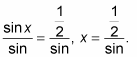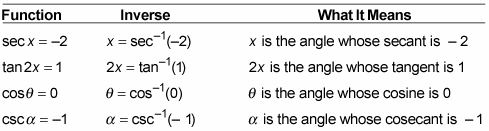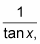##### Trigonometry Workbook For DummiesYou use inverse trigonometry functions to solve equations such as sin x = 1/2, sec x = –2, or tan 2x = 1. In typical algebra equations, you can solve for the value of x by dividing each side of the equation by the coefficient of the variable or by adding the same thing to each side, and so on. But you can’t do either with the function sin x = 1/2.

Would it make sense to divide each side by sine? “Out, out thou sine!” Here’s what you’d get:Goodness, no! That’s silly. In fact, it’s a sin. (Sorry!)

To find the inverse of an equation such as sin x = 1/2, solve for the following statement: “x is equal to the angle whose sine is 1/2.” In trig speak, you write this statement as x = sin1(1/2). The notation involves putting a –1 in the superscript position. Here are some more examples of trig equations with their corresponding inverses and the translation.You’ve undoubtedly seen and used the exponent –1 in math expressions before now. But that exponent does a different kind of job for inverse trig functions and relations. The notation for an inverse trig relation such as sin1 x means that you want an inverse for the expression, not the reciprocal. If you wantyou have to write (tan x)–1 with parentheses. The –1 exponent is where the exponential notation for trig functions makes a big exception.

When raising trig functions to a power, sin2 x = (sin x)2 and cos4 x = (cos x)4, but tan1 x means the inverse function, not raising tan x to the –1 power.

Inverses of trig functions have an alternate notation that avoids the confusion over what the –1 superscript means: the arc name. Another way of saying sin1 x is arcsin x. The inverse cosine is cos–1 x, or arccos x. The other inverse functions are arctan x, arccsc x, arcsec x, and arccot x. This notation is longer and sometimes awkward to write out, so the original superscript notation is often preferable. You’ll see inverse functions written both ways, though.